# Operating Ratio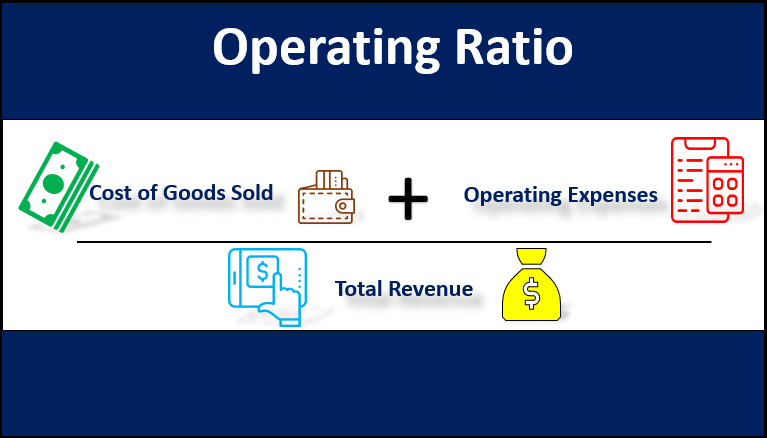## What is the Operating Ratio?

The term “operating ratio” refers to the metric that measures the ability of a company to manage its Operating expenses in a normal business set-up. In other words, it gauges the efficiency of a company’s management to keep its operating expenses low while generating revenue. The OR is also known as expenses-to-sales ratio and the expenses can either be individual expense or group of expenses.

In fact, lower the ratio means higher is the efficiency of the company to generate dollar versus the operating expenses which will leave a portion of the sales to result in higher profit margin or higher returns for the investors. On the other hand, a higher ratio results in a less favorable situation as it indicates lower profitability and so lower returns for the investors.

Formula

The formula for an OR can be derived by dividing the sum of the cost of goods sold and the operating expenses by the total revenue of the subject company. Mathematically, it is represented as,

Operating Ratio = (Cost of Goods Sold + Operating Expenses) / Total Revenue

Here, the cost of goods sold includes all the direct cost of manufacturing such as the cost of raw materials and labor wages, while operating expenses include all the indirect cost of production such as selling & distribution expenses, office & administration expenses, etc.

Cost of Goods Sold = Cost of Raw Materials + Labour Wages

Operating Expenses = Selling & Distribution Expenses + Office & Administration Expenses

### Examples of Operating Ratio (With Excel Template)

Let’s take an example to understand the calculation of the OR in a better manner.

You can download this Operating Ratio Excel Template here – Operating Ratio Excel Template

#### Example – #1

Let us take the example of a company named ZXC Ltd to illustrate the concept of operating ratio. As per the latest income statement, the following information is available: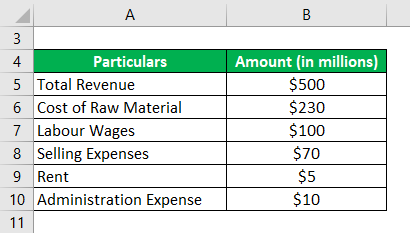Solution:

Cost of Goods Sold is calculated by using the formula given below

Cost of Goods Sold = Cost of Raw Materials + Labour Wages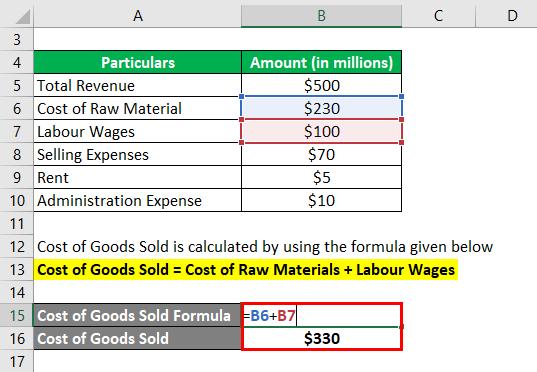• Cost of Goods Sold = \$230 million + \$100 million
• Cost of Goods Sold= \$330 million

Operating Expenses is calculated by using the formula given below

Operating Expenses= Selling Expenses + Rent + Administration Expense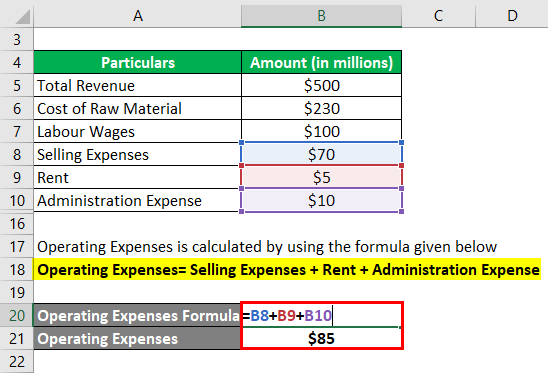• Operating Expenses = \$70 million + \$5 million + \$10 million
• Operating Expenses = \$85 million

Operating Ratio is calculated by using the formula given below

Operating Ratio = (Cost of Goods Sold + Operating Expenses) / Total Revenue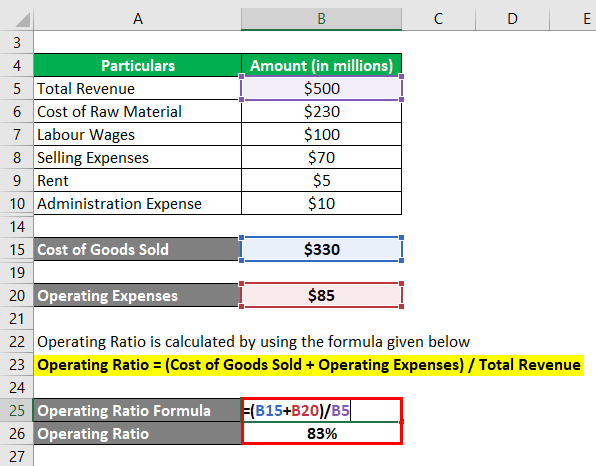• OR = (\$330 million + \$85 million) / \$500 million
• OR = 83%

Therefore, the operating ratio of ZXC Ltd is 83% for the latest year.

#### Example – #2

Let us take the example of Apple Inc. and calculate its operating ratio for the year 2018. As per the annual report for the year ending on September 29, 2018, the following information is available: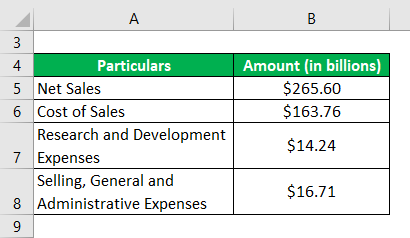Solution:

Operating Expenses is calculated by using the formula given below

Operating Expense = Research and Development Expenses + Selling, General and Administrative Expenses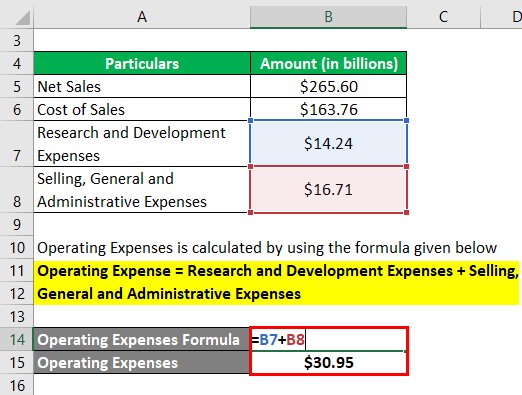• Operating Expense = \$14.24 billion + \$16.71 billion
• Operating Expense = \$30.95 billion

It can be calculated by using the formula given below

Operating Ratio = (Cost of Goods Sold + Operating Expenses) / Total Revenue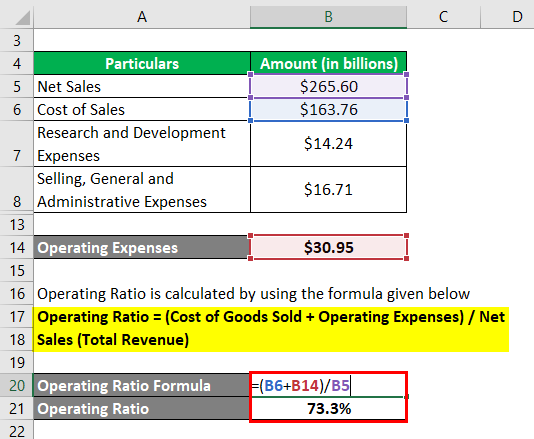• OR = (Cost of Sales + Operating Expenses) / Net Sales
• OR = (\$163.76 billion + \$30.95 billion) / \$265.60 billion
• OR = 73.3%

Therefore, the operating ratio of Apple Inc. stood at 73.3% during the year 2018.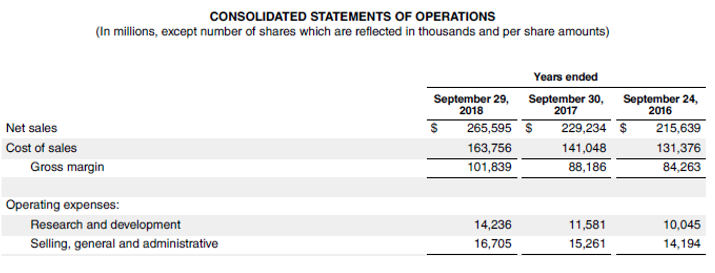Source: d18rn0p25nwr6d.cloudfront.net

#### Example – #3

Let us take the example of Walmart Inc. and calculate its operating ratio for the year 2018. As per the annual report for the year ending on January 31, 2018, the following information is available: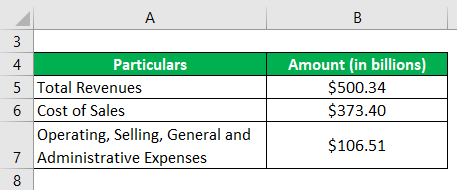Solution:

Operating Ratio is calculated by using the formula given below

Operating Ratio = (Cost of Goods Sold + Operating Expenses) / Total Revenue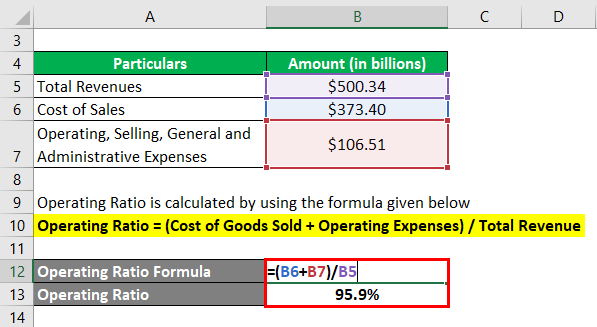• OR = (\$373.40 billion + \$106.51 billion) / \$500.34 billion
• OR = 95.9%

Therefore, the operating ratio of Walmart Inc. stood at 95.9% during the year 2018.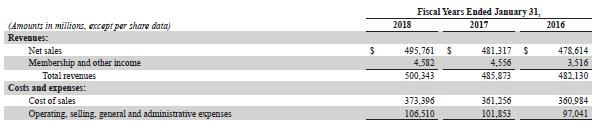Source: s2.q4cdn.com

• The ratio is a great indicator of the operational efficiency of a company’s management. A lower value of the ratio indicates better operational acumen of the management.
• Higher profitability is the eventual goal of any company and this ratio provides a measure of the same.

• Since the ratio doesn’t take into account the effect of interest expense, as such companies with high debt might get away with this ratio. So, two companies with similar operating ratios can have a different capital structure.
• The ratio doesn’t make much of a sense if seen in isolation. So, it should be either be viewed in comparison to its peers or be monitored across the period to check trends.

### Conclusion

So, operating ratio can be a very useful tool to measure the efficiency of a company when compared to peers or insights that can be drawn when monitored over a period of time to see how well the company management is performing.

### Recommended Articles

This is a guide to the Operating Ratio. Here we discuss how to calculate the Operating Ratio using its formula with examples and a downloadable Excel template. You can also go through our other suggested articles to learn more –

1. Cash Reserve Ratio
2. Solvency Ratio
3. Equity Ratio
4. Defensive Interval Ratio

The post Operating Ratio appeared first on EDUCBA.

This post first appeared on Free Online CFA Calculator Training Course | EduCB, please read the originial post: here